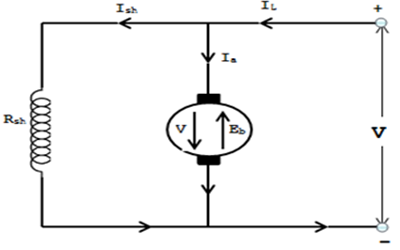# DC Motor Voltage Equation and Power Equation### Voltage Equation:

Consider a shunt wound motor shown in Fig.
Let,         V = applied voltage
Eb = back e.m.f.
Ia = armature current
Ra = armature resistance

Meanwhile back e.m.f. Eb acts in reverse to the applied voltage V, the net voltage through the armature circuit is V-Eb. Now the armature current Ia is given by;
Ia = V-Eb/Ra
V = Eb + Ia Ra       ====> (1)
This is known as voltage equation of the d.c. motor.

### Power Equation:

If the above Eqn. (1) is multiplied with Ia throughout, we get,
VIa = Eb Ia + I2a Ra

This is said to be a power equation of the d.c. motor.

Where,
VIa = armature input (electric power supplied to armature)
EbIa = armature output (power developed by armature)
Ia Ra = armature Cu loss (electric power wasted in armature)

Therefore out of the armature input, a small portion (about 5%) is wasted as I2aRa and the remaining portion EbIa is changed into mechanical power within the armature.0 CommentsComments